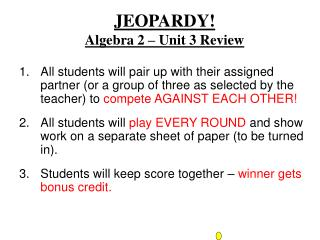DownloadDownload PresentationJEOPARDY! Algebra 2 – Unit 3 Review

# JEOPARDY! Algebra 2 – Unit 3 Review

Download Presentation## JEOPARDY! Algebra 2 – Unit 3 Review

- - - - - - - - - - - - - - - - - - - - - - - - - - - E N D - - - - - - - - - - - - - - - - - - - - - - - - - - -
##### Presentation Transcript

1. JEOPARDY! Algebra 2 – Unit 3 Review • All students will pair up with their assigned partner (or a group of three as selected by the teacher) to compete AGAINST EACH OTHER! • All students will play EVERY ROUND and show work on a separate sheet of paper (to be turned in). • Students will keep score together – winner gets bonus credit.

2. A Picture Is Worth A Thousand Words Word World! 3’s Company The Basics 100 200 200 100 300 200 500 300 500 400 1000 500 700 We’re Done! Good Luck on the Test!!

3. 100 Solve for x and y: x + 2y = 11 2x – y = 2

4. x + 2y = 11 x + 2y = 11 (2x – y = 2)  2 4x – 2y = 4 5x = 15  x = 3 100 x = 3, y = 4 or (3, 4)

5. 200 Solve for x and y: x = 2y – 3 y – 3x = –1

6. x = 2y – 3 y – 3x = –1 y – 3(2y – 3) = –1 -5y + 9 = -1 y = 2 x = 2(2) – 3 = 1 x = 1, y = 2 or (1, 2) 200

7. 400 Solve for x and y: 4x – 3y = 5 8x – 6y = 12

8. ( 4x – 3y = 5 )  –2 –8x + 6y = –10 8x – 6y = 12 8x – 6y = 12 0 = 2 400 Since 0 = 2 can NEVER be true … “NO SOLUTION” !!!

9. 200 The sum of two numbers is 24. The first number is 4 less than the second number. Find the two numbers.

10. Let x = first number Let y = second number x + y = 24 x = y – 4 x = 10, y = 14 200

11. 300 Brandon has a pocket full of nickels and dimes. If he has 20 coins worth \$1.30 in his pocket, how many of each coin does he have?

12. 300 Let N = # of nickels Let D = # of dimes ( N + D = 20 )  –5 ( .05N + .10D = 1.30 )  100 N = 14, D = 6

13. 500 Twyla is taking her friends to a concert. Tickets cost \$8 for general admission and \$10 for reserved seating. If Twyla buys 12 tickets for a total of \$102, how many of each kind of ticket did she buy?

14. 500 Let G = # of general tickets Let R = # of reserved tickets ( G + R = 12 )  –8 8G + 10R = 102 G = 9, R = 3

15. 700 The perimeter of a rectangle is 44. If the length is 6 more than the width, then find the length and the width.

16. Let L = length, W = width 2L + 2W = 44 L = W + 6 Substitute to get … 2(W + 6) + 2w = 44 L = 14, W = 8 700

17. 100 Graph the following and identify the solution: y = 2x – 8 2y + x = 4

18. y = 2x – 8 2y + x = 4 y = –½ x + 2 100 (4, 0)

19. 300 Graph the following and identify the solution: y  3x – 3 y  –2x + 4

20. y  3x – 3 y  –2x + 4 300

21. 500 Graph the following and identify the solution: y  –5 x  2 y  x + 4

22. y  –5 x  2 y  x + 4 500

23. 200 Solve for x, y and z: 3x + 5y + z = 5 x – 2y = –9 2x = –10

24. 3x + 5y + z = 5 x – 2y = –9 2x = –10 200 x = –5 y = 2 z = 10

25. 500 Solve for x, y, and z: 2x – y = 2 2y – z = 8 3x + z = 9

26. 2x – y = 2 2y – z = 8 4x – 2y = 4 3x + z = 9 3x + 2y = 17 7x = 21 500 x = 3 y = 4 z = 0

27. 1000 Solve for x, y and z: x – y + z = –2 3x + 2y + z = 6 2x + 3y – 2z = 10

28. x – y + z = –2 3x + 2y + z = 6 2x + 3y – 2z = 10 1000 x = 1 y = 2 z = –1